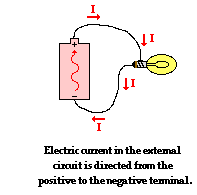# Year 8 Electricity

20 Questions | Total Attempts: 3621SettingsYear 8 Electricity Test

Related Topics
• 1.
Electric current in a/an ____________________ circuit travels in MORE THAN ONE path.
• A.

Series

• B.

Wire

• C.

Parallel

• D.

Connected

• 2.
A drawing that uses symbols to show how the parts of an electric circuit are connected is a/an _____________________________.
• A.

Nichrome wire

• B.

Electric diagram

• C.

Parallel circuit

• D.

Circuit diagram

• 3.
Electric current in a/an _____________________ circuit travels in ONE path.
• A.

Series

• B.

Parallel

• C.

Electric

• D.

Wire

• 4.
A material through which electric current CANNOT pass easily is a/an ____________________.
• A.

Tester

• B.

Insulator

• C.

Receptor

• D.

Conductor

• 5.
A material through which electric current CAN pass easily in a/an ______________________/
• A.

Tester

• B.

Insulator

• C.

Receptor

• D.

Conductor

• 6.
• A.

Filament

• B.

Nichrome wire

• C.

Circuit

• D.

Wire

• 7.
In order for a circuit to work, the path must be __________________.
• A.

Series

• B.

Paralled

• C.

Open

• D.

Closed

• 8.
A/An _____________________ is used to turn the electricity in a current on or off.
• A.

Battery

• B.

Switch

• C.

Conductor

• D.

Insulator

• 9.
• A.

Electric current

• B.

Circuit tester

• C.

Battery

• D.

Wire

• 10.
A metal strand used to connect electrical devices in a circuit is a/an ________________________.
• A.

Battery

• B.

Switch

• C.

Filament

• D.

Wire

• 11.
A power source than can produce an elecctric current as a result of a chemical change in it is a/an ______________________.
• A.

Circuit

• B.

Wire

• C.

Battery

• D.

Filament

• 12.
Which of the following is a good conductor?
• A.

Plastic

• B.

Glass

• C.

Iron

• D.

Wood

• 13.
Which of the following not a conductor of electricity?
• A.

Iron

• B.

Copper

• C.

Plastic

• D.

Graphite

• 14.
• A.

• B.

• C.

Energy source, wire, switch

• D.

Battery, wire, switch

• 15.
What is resistance?
• A.

Opposition to current

• B.

Energy from battery

• C.

An increase in current

• D.

An increase in voltage

• 16.
Which device is used to measure current?
• A.

Ammeter

• B.

Voltmeter

• C.

Resistor

• D.

Circuit beaker

• 17.
Which device is used to measure voltage?
• A.

Ammeter

• B.

Voltmeter

• C.

Resistor

• D.

Circuit beaker

• 18.
• A.

Open circuit

• B.

Closed circuit

• C.

Short circuit

• D.

Direct circuit

• 19.
What is the function of a fuse?
• A.

Another name for a switch

• B.

A protective device to slow down the current

• C.

A wire with high resistance to prevent current from passing through

• D.

A protective device for breaking an electric circuit.

• 20.
Which is the correct unit for current?
• A.

Ohms

• B.

Volts

• C.

Watts

• D.

Amperes